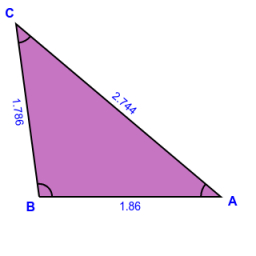# Magnitudes 64704

The triangle ABC determines the size of the sides a and b and the magnitudes of the interior angles β and γ, given c = 1.86 m, the line on the side c is 2.12 m, and the angle alpha is 40 ° 12 '.

a =  1.7865 m
b =  2.7436 m
β =  97.576 °
γ =  42.224 °

### Step-by-step explanation:

Our quadratic equation calculator calculates it.

Try calculation via our triangle calculator.Did you find an error or inaccuracy? Feel free to write us. Thank you!

Tips for related online calculators
Are you looking for help with calculating roots of a quadratic equation?
Need help calculating sum, simplifying, or multiplying fractions? Try our fraction calculator.
Do you have a linear equation or system of equations and looking for its solution? Or do you have a quadratic equation?
Do you know the volume and unit volume, and want to convert volume units?
The Pythagorean theorem is the base for the right triangle calculator.
Do you want to convert time units like minutes to seconds?
Cosine rule uses trigonometric SAS triangle calculator.